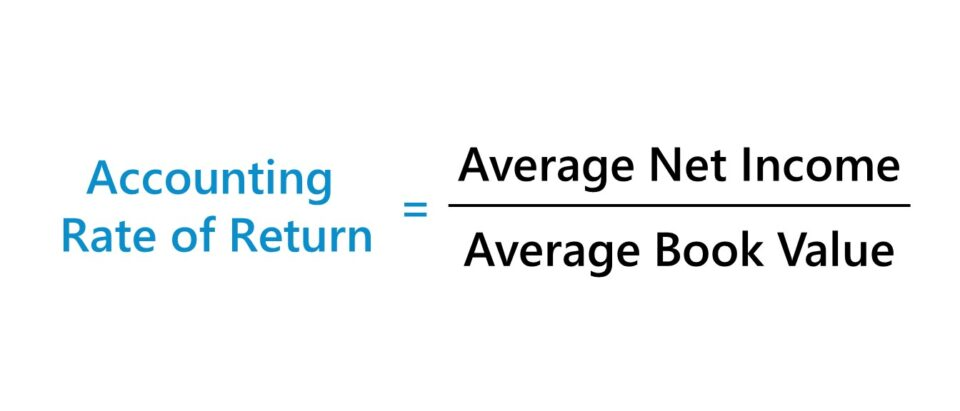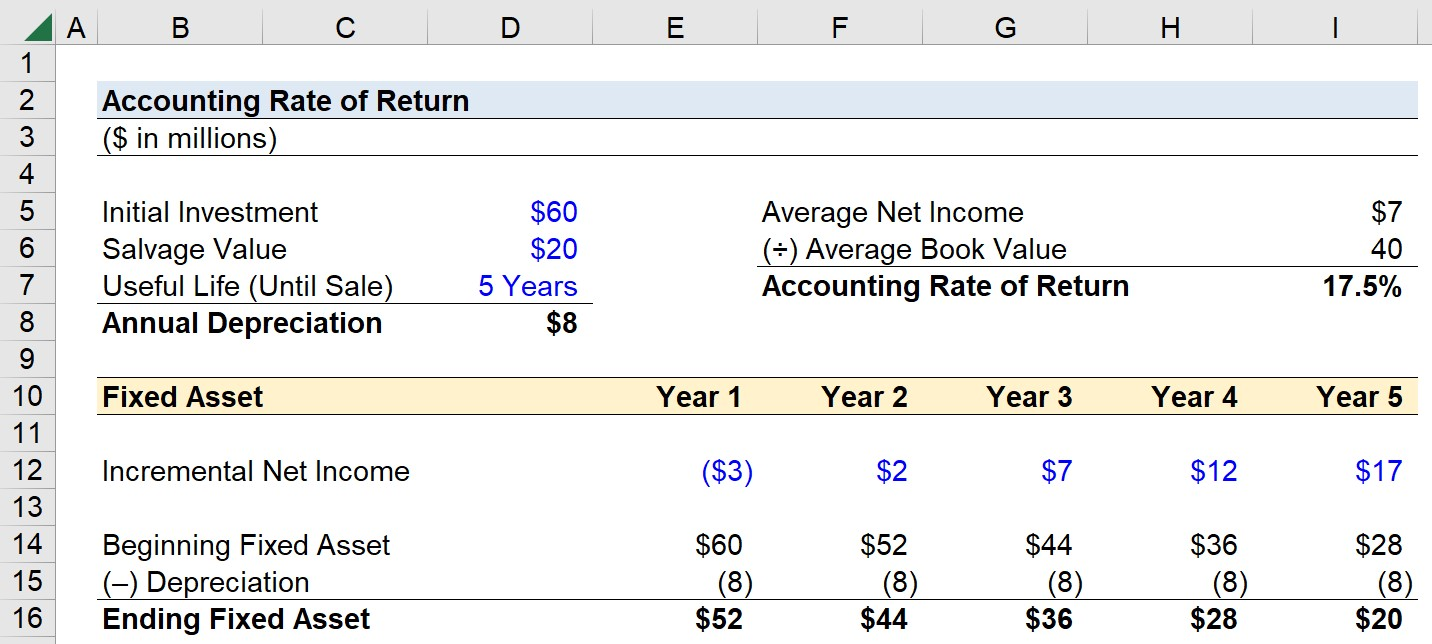# Accounting Rate of Return

Guide to Understanding Accounting Rate of Return (ARR)## How to Calculate Accounting Rate of Return (Step-by-Step)

In capital budgeting, the accounting rate of return, otherwise known as the “simple rate of return”, is the average net income received on a project as a percentage of the average initial investment.

By comparing the average accounting profits earned on a project to the average initial outlay, a company can determine if the yield on the potential investment is profitable enough to be worth spending capital on.

If the project generates enough profits that either meet or exceed the company’s “hurdle rate” – i.e. the minimum required rate of return – the project is more likely to be accepted (and vice versa).

The primary drawback to the accounting rate of return is that the time value of money (TVM) is neglected, much like with the payback period. Hence, the discounted payback period tends to be the more useful variation.

## Accounting Rate of Return Formula

The formula to calculate the accounting rate of return is as follows.

Accounting Rate of Return = Average Net Income ÷ Average Book Value

On the income statement, net income (i.e. the “bottom line”) is a company’s accrual-based accounting profit after all operating costs (e.g. COGS, SG&A and R&D) and non-operating costs (e.g. interest expense, taxes) are deducted.

The standard conventions as established under accrual accounting reporting standards that impact net income, such as non-cash expenses (e.g. depreciation and amortization), are part of the calculation.

The average book value refers to the average between the beginning and ending book value of the investment, such as the acquired fixed asset.

Average Book Value = (Beginning Book Value + Ending Book Value) ÷ 2

Where:

• Beginning Book Value: The beginning book value is straightforward, as it refers to the initial outlay on the date of purchase.
• Ending Book Value: On the other hand, the ending book value is the residual book value, i.e. the initial investment net of the accumulated depreciation until the end of the fixed asset’s useful life (and date of sale).

## Accounting Rate of Return Calculator – Excel Model Template

We’ll now move on to a modeling exercise, which you can access by filling out the form below.Submitting ...

## Accounting Rate of Return Calculation Example (ARR)

Suppose you’re tasked with calculating the accounting rate of return from purchasing a fixed asset using the following assumptions.

• Initial Investment = \$60 million
• Salvage Value (or Scrap Value) = \$20 million
• Useful Life (Until Sale) = 5 Years

Given those figures, we can determine the depreciation is \$8 million per year.

• Annual Depreciation = (\$60 million – \$20 million) ÷ 5 Years = \$8 million

The incremental net income generated by the fixed asset – assuming the profits are adjusted for the coinciding depreciation – is as follows.

• Incremental Net Income, Year 1 = (\$3 million)
• Incremental Net Income, Year 2 = \$2 million
• Incremental Net Income, Year 3 = \$7 million
• Incremental Net Income, Year 4 = \$12 million
• Incremental Net Income, Year 5 = \$17 million

Next, we’ll build a roll-forward schedule for the fixed asset, in which the beginning value is linked to the initial investment, and the depreciation expense is \$8 million each period.

• Ending Fixed Asset, Year 1 = \$52 million
• Ending Fixed Asset, Year 2 = \$44 million
• Ending Fixed Asset, Year 3 = \$36 million
• Ending Fixed Asset, Year 4 = \$28 million
• Ending Fixed Asset, Year 5 = \$20 million

The ending fixed asset balance matches our salvage value assumption of \$20 million, which is the amount the asset will be sold for at the end of the five-year period.

With the two schedules complete, we’ll now take the average of the fixed asset’s net income across the five-year time span and divide it by the average book value.

The total profit from the fixed asset investment is \$35 million, which we’ll divide by five years to arrive at an average net income of \$7 million.

• Average Net Income = \$35 million ÷ 5 Years = \$7 million

The average book value is the sum of the beginning and ending fixed asset book value (i.e. the salvage value) divided by two.

• Average Book Value = (\$60 million + \$20 million) ÷ 2 = \$40 million

In conclusion, the accounting rate of return on the fixed asset investment is 17.5%.

• Accounting Rate of Return = \$7 million ÷ \$40 million = 17.5%Step-by-Step Online Course

### Everything You Need To Master Financial Modeling

Enroll in The Premium Package: Learn Financial Statement Modeling, DCF, M&A, LBO and Comps. The same training program used at top investment banks.